Here, we record all of the formulas mentioned in our guide:

# General

Rule 1: Shapes and figures are not necessarily drawn to scale.

Rule 2: Shapes and figures do show the relative position of different objects.

Rule 3: Co-ordinate systems and the number line are to scale.

# Polygons

Regular Polygon: Regular polygons have sides of equal length and angles of equal degree.

Angles of a Regular Polygon
If we have a regular polygon ofsides, each angle isdegrees.

# Triangles

Triangle Area Formula:Angles of a Triangle Sum to 180: For any triangle, the sum of its interior angles is 180 degrees.

Triangle Inequality: The sum of the length of any two sides of a triangle is greater than the length of its third side.

Pythagorean Theorem: For any right triangle whereare the legs of the triangle andis the hypotenuse,Reverse Pythagorean Theorem: If a triangle has side lengthssuch that, then the triangle is a right triangle.

30-60-90 Right Triangle: For any right triangle with angles of 30, 60, and 90 degrees, the side lengths have the following ratio: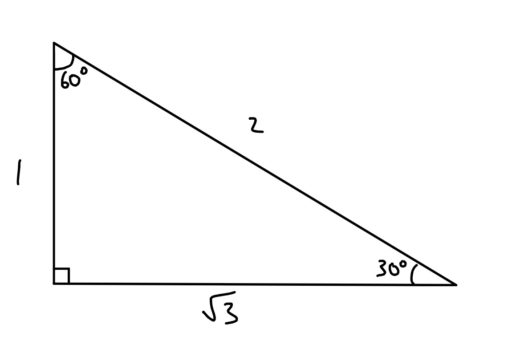45-45-90 Right Triangle: Any right triangle with angles of 45, 45 and 90 degrees will have the following side lengths (whereis some fixed number):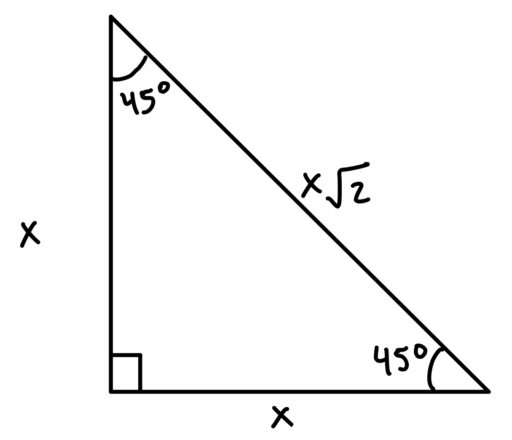Equilateral Triangles: All sides have the same length.

Isosceles Triangles: Two sides have the same length as each other; the third has a different length.

Equal Sides Have Equal Angles: In an isosceles triangle, the angles opposite from the sides of equal length must have equal degree.

Scalene Triangles: All of the sides are of different lengths.

Acute Triangles: All angles are less than 90 degrees.

Right Triangles: One angle is exactly 90 degrees.

Obtuse Triangles: One angle is more than 90 degrees.

Similar: Two triangles are similar if their angles have the same values.

Similar Triangles Share Proportions: If two triangles are similar, then there is some constant ratio you can multiply the sides of one triangle by in order to get the sides of the other triangle.

Congruent: Two triangles are congruent if their angles are the same and their sides are the same length.

# Circles

Circle: A 2D shape whose points are always the same distance from some central point.

Center: The center of a circle is the point within the circle from which every point of the circle is the same distance. We often label the center and use it to identify the circle (e.g. “the circle whose center is at A”).

Radius: Any line from the center of a circle to any point on the circle. We often use the variablefor the length of the radius.

Area of a Circle: The area of a circle with radiusisDiameter: Any line that connects two points on the circle and the center of the circle. We often use the variablefor the length of the diameter.

Diameter Formula: For a circle with radius, the diameterCircumference: The distance around the circle.

Circumference Formula: The circumference of a circle with a radiusisChord: Any line segment that connects two points on a circle.

Diameter is Longest Chord: The diameter of a circle is also a chord of the circle and, in fact, it is the longest chord on a circle.

Arc: Any portion of a circle’s circumference located between two points on the circle.

Sector: A portion of the circle enclosed by two radii and an arc.

Tangent: A straight line that touches a curve or circle at a single point

Point of Tangency: The point at which a tangent line touches a curve. Note that the tangent line (in red below) will be perpendicular to any line connecting the center and the point of tangency.

Inscribed: Circles can be inscribed within other shapes. An inscribed circle is the largest possible circle that can be drawn wholly within another shape. For example: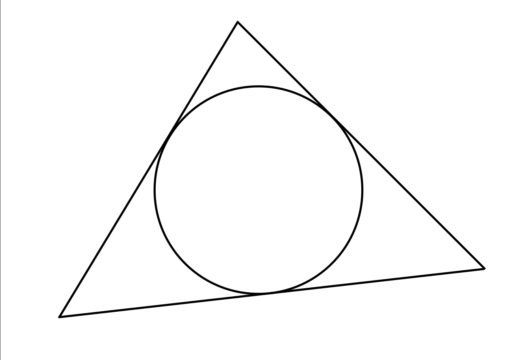Circumscribed: Circles can also be drawn around other shapes. A circumscribed circle is the smallest possible circle that can be drawn wholly outside of another shape. For example: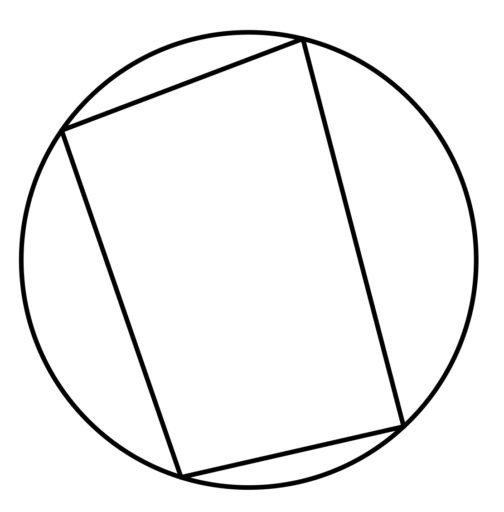Concentric Circles: Two circles are concentric if they share the same center.

Rectangles: four sides connected by four 90-degree angles

Rectangle Area Formula:Squares: four sides of equal length connected by four 90-degree angles.

Square Area Formula:Parallelograms: four-sided figure where opposite sides are parallel

Parallelogram Area Formula:Rhombus: a parallelogram with equal sides

Rhombus Area Formula:Trapezoids: four-sided figure with one pair of parallel opposing sides

Trapezoid Area Formula:Quadrilaterals Have 360 Degrees: The sum of the angles in a quadrilateral is 360.

Perimeter of a Square or Rectangle: In the following diagrams, the perimeter of the square isand the perimeter of the rectangle isPerimeter of a Rhombus: Since a rhombus is defined by having four sides of equal length, the perimeter of the below rhombus is justPerimeter of a Parallelogram
Remember that the parallel sides of a parallelogram have the same length. Thus, for the below parallelogram: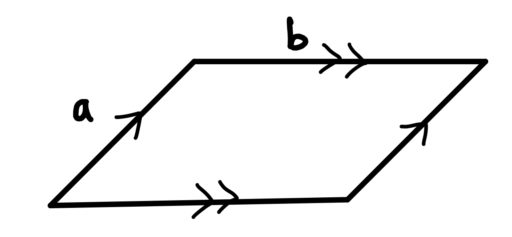the perimeter is just.

Perimeter of a Trapezoid: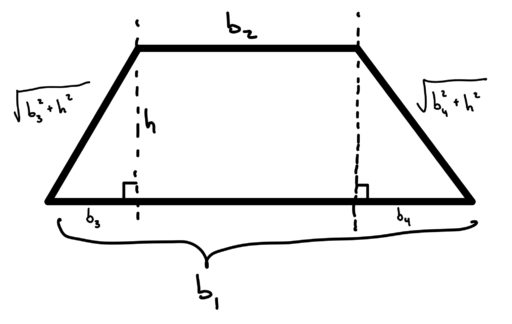The perimeter is:# 3D Shapes

Rectangular Solid: A 3D shape with six faces which are all rectangles placed perpendicularly to one another.

Volume of a Rectangular Solid: For the following rectangular solid,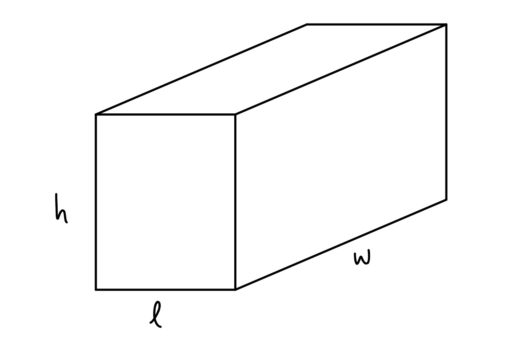its volume is equal toSurface Area of a Rectangular Solid: For the following rectangular solid,its surface area is equal toCube: a cube is a rectangular solid whose six sides are all squares.

Volume of a Cube: The volume of a cube is.

Surface Area of a Cube: The surface area of a cube is.

Circular Cylinder: A 3D shape that has a circle for both bases and a perpendicular line connecting the center of those bases.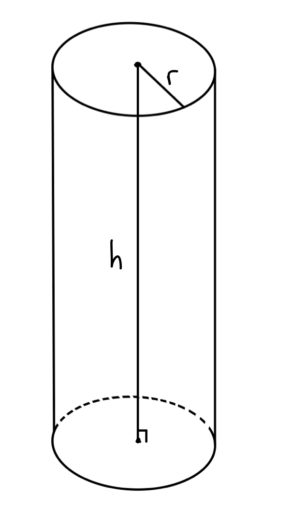Volume of a Circular Cylinder: The volume of a right circular cylinder with a radius ofand a height ofisSurface Area of a Circular Cylinder: The surface area of a circular cylinder with a radius ofand a height ofis# Angles

Line: a straight line that continues in both directions without end.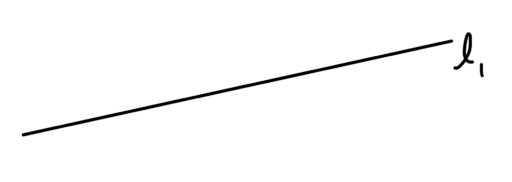Angle: a measure of how much you would need to turn one line to make them part of the same line

Lines Have 180 Degrees: The angle formed by a single line has 180 degrees.

Circles Have 360 Degrees: The angle formed by a circle is 360 degrees.

Parallel Lines: Parallel lines go in the same direction and therefore never intersect. We often denote that linesandare parallel by writing, as in the below diagram: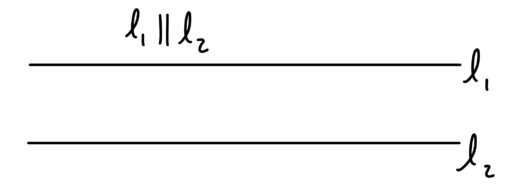Perpendicular Lines: Perpendicular lines intersect at a 90 degree angle. We denote two lines as perpendicular by writing: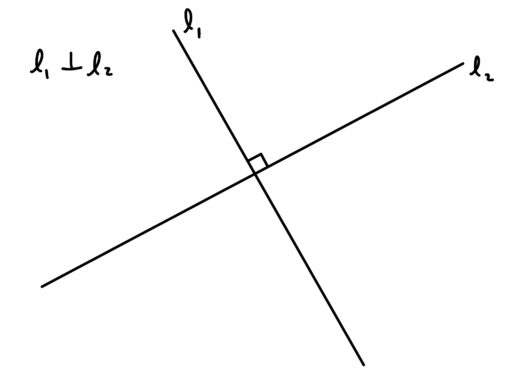Vertical Angles: Two angles formed by the same lines are vertical angles if they are on opposite sides of the point of intersection between the two lines.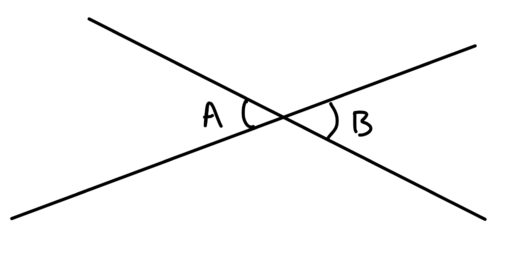Interior Angles: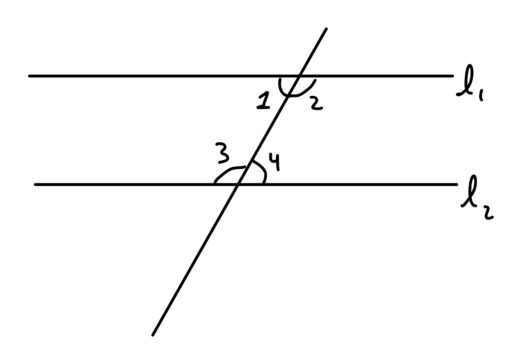In the above diagram,Exterior Angles: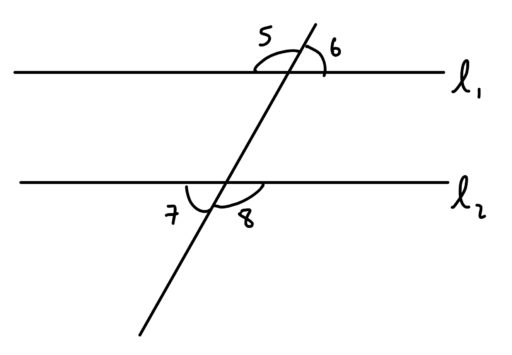In the above diagram,Corresponding Angles: Angles who are in the same relative position across parallel lines are equal to one another. For example,in the below: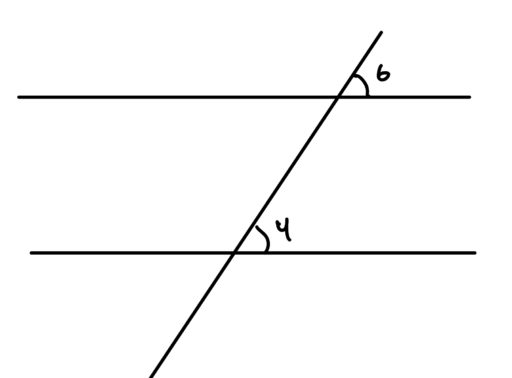Triangles have 180 degrees: The angles of a triangle add up to 180 degrees.

Central Angle: A central angle is an angle located at the center of a circle with endpoints on the circumference of that circle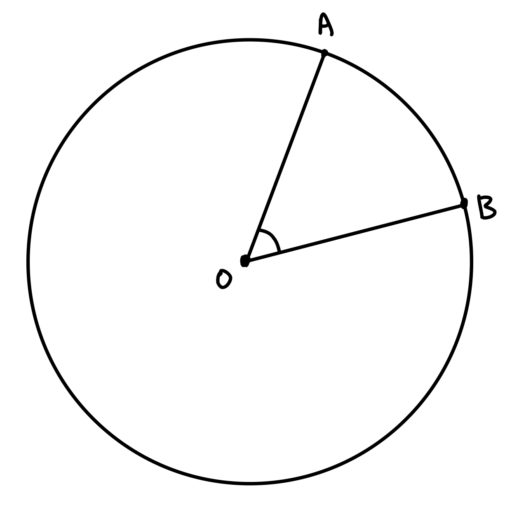Central Angles and Arcs: In the following diagram: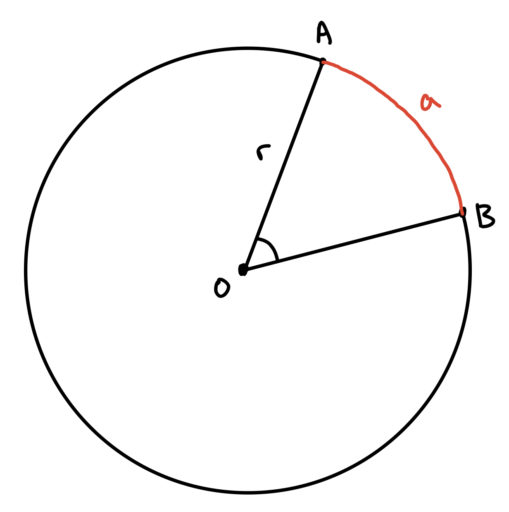The degree of angle AOB =Circumference Angle Theorem: In the following circle with radius: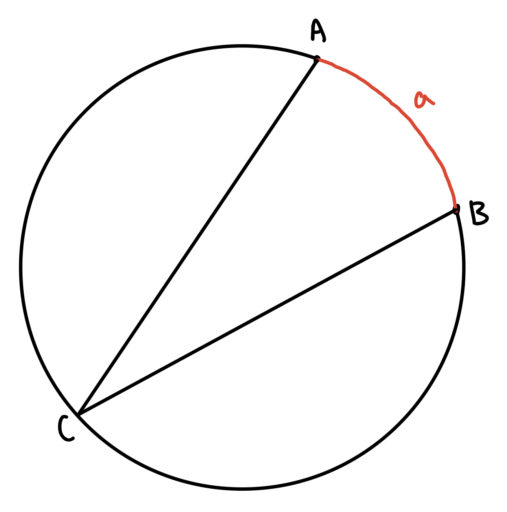The degree of angle ACB =.